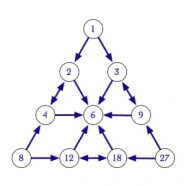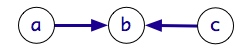# Iconic ArithmeticThe Image is of the Greek Tetraktys, the philosophical/metaphysical icon of the Pythagorean School.  Little wonder the Greeks saw whole numbers as magical, cause the Tetraktys exposes so many tightly coupled numerical relations.  Each set of arrows in the image shows the geometric mean.The physical principles and the common sense activities of arithmetic were lost at the turn of the twentieth century when mathematics committed to the abstract Rules of Symbolic Algebra.  These symbolic rules were then propagated in textbooks and classrooms as what arithmetic is and as what we must know in order to be able to “do” arithmetic.  The abstract rules of symbol manipulation that are currently taught in all classrooms are not how arithmetic works, they are how typographical symbols must work when those symbols are used to convey the simple physical principles of arithmetic. The arithmetic used by people is based in physical behavior, not in abstract symbols.

Iconic arithmetic reintroduces the Principles of Arithmetic that have been in use since the dawn of civilization. The intuitive Principles of Iconic Arithmetic define how arithmetic works. The Principles are easily understood, intuitive actions that are currently being extinguished by math education as it embraces abstract rather than concrete mathematics.

The Additive Principle A sum looks like its parts.

The Multiplicative Principle A product matches units with groups.

These Principles reaffirm that arithmetic is based in physical activity. Addition is simply putting units together.  Multiplication is replacing units by groups.

Addition x + y  ==> Put x and y together (in the same container).

Multiplication x * y  ==> Put y into x (substitute y for each unit of x).

For example:

••• + •• = ••••• Put ••• together with ••

••• x •• = •• •• •• Put •• in place of each unit of •••

Students who find the abstract rules of symbolic algebra (rules like arity, commutativity and associativity) confusing and intolerable are exercising good judgment.  Math classes currently expect students to arbitrarily replace their organic wisdom and experience with disconnected symbol juggling rules. The solution is to use a representation of elementary arithmetic that is more closely anchored to reality.